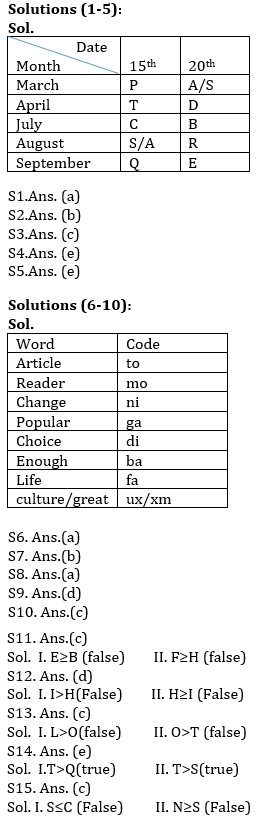Latest Banking jobs   »   Reasoning Ability Quiz For IBPS Clerk...

# Reasoning Ability Quiz For IBPS Clerk Prelims 2021- 24th July

Directions (1-5): Study the following information carefully to answer the given questions:
Ten persons A, B, C, D, E, P, Q, R, S and T are going for vacations on two different dates i.e. 15th and 20th of five months viz. January, April, July, August and November.
Four persons goes between C and E, who goes after C. T goes on vacation on one of the months before C. Both C and T goes in the same date. Two persons goes between B and T. Q goes on vacation in one of the months which has 30 days but not on 20th. D goes immediately after T. Four persons goes between A and S. S goes on vacation before R and after P.

Q1. Who among the following goes on 15th April?
(a) T
(b) C
(c) Q
(d) B
(e) None of these

Q2. Who among the following goes immediately before B?
(a) Q
(b) C
(c) D
(d) T
(e) None of these

Q3. Who among the following goes on 20th August?
(a) P
(b) A
(c) R
(d) S
(e) None of these

Q4. A goes on vacation on which among the following date?
(a) 15th January
(b) 20th January
(c) 15th August
(d) 20th August
(e) Cannot be determined

Q5. How many persons goes before S?
(a) One
(b) Three
(c) Five
(d) Six
(e) Either (a) or (d)

Directions (6-10): Study the following information and answer the given questions:
In a certain code language
‘change article enough popular choice’ is written as “to ga di ba ni”
‘choice article culture change great’ is written as “ux to ni di xm”
‘reader popular change’ is written as “ga ni mo”.
‘article culture great life’ is written as ‘ux xm fa to’.

Q6. What does the code ‘ga’ stand for in the given code language?
(a) popular
(b) choice
(c) enough
(d) article
(e) None of these

Q7. What is the code for ‘life’ in the given code language?
(a) to
(b) fa
(c) xm
(d) ux
(e) None of these

Q8. What is the code “fa mo ba” stand for in the given code language?
(b) great enough life
(e) None of these

Q9. What may the code ‘ux mo di’ stand for in the given code language?
(a) culture great choice

Q10. What is the code for ‘change’ in the given code language?
(a) ba
(b) di
(c) ni
(d) ga
(e) None of these

Directions (11-15): In these questions, a relationship between different elements is shown in the statements. The statements are followed by two conclusions. Give answer

Q11.
Statement: H<B; H≥D>E; G≥F>B
Conclusion: I. E≥B II. F≥H
(a) if either conclusion I or II is true.
(b) if only conclusion I is true.
(c) if neither conclusion I nor II is true.
(d) if only conclusion II is true.
(e) if both conclusions I and II are true.

Q12.
Statement: K<J; D≤S=J; I>S; H≥J
Conclusion: I. I>H II. H≥I
(a) if both conclusion I and II are true.
(b) if neither conclusion I nor II is true.
(c) if only conclusion I is true.
(d) if either conclusion I or II is true.
(e) if only conclusion II is true.

Q13.
Statement: J<L; K≥S; K≥O; S>L≥T
Conclusion: I. L>O II. O>T
(a) if only conclusion II is true.
(b) if either conclusion I or II is true.
(c) if neither conclusion I nor II is true.
(d) if only conclusion I is true.
(e) if both conclusions I and II are true.

Q14.
Statement: T>N; A>S>Q; A<N
Conclusion: I.T>Q II. T>S
(a) if only conclusion II is true.
(b) if only conclusion I is true.
(c) if neither conclusion I nor II is true.
(d) if either conclusion I or II is true.
(e) if both conclusions I and II are true.

Q15.
Statement: B≤C; B≤D; C=N; D<S
Conclusion: I. S≤C II. N≥S
(a) if only conclusion II is true.
(b) if either conclusion I or II is true.
(c) if neither conclusion I nor II is true.
(d) if only conclusion I is true.
(e) if both conclusions I and II are true.

Solutions